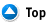Shapes Formula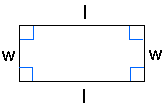Rectangle: Area = Length X Width A = lw Perimeter = 2 X Lengths + 2 X Widths P = 2l + 2w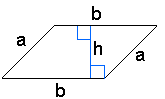Parallelogram Area = Base X Height a = bh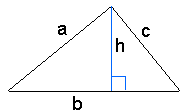Triangle Area = 1/2 of the base X the height a = 1/2 bh Perimeter = a + b + c (add the length of the three sides)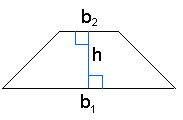Trapezoid Perimeter = a + b1 + b2 + c P = a + b1 + b2 + c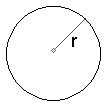Circle The distance around the circle is a circumference. The distance across the circle is the diameter (d). The radius (r) is the distance from the center to a point on the circle. (Pi = 3.14) d = 2r c = pd = 2 pr A = pr2 (p=3.14)Rectangular Solid Volume = Length X Width X Height V = lwh Surface = 2lw + 2lh + 2whPrisms Volume = Base X Height v=bh Surface = 2b + Ph (b is the area of the base P is the perimeter of the base)Cylinder Volume = pr2 x height V = pr2 h Surface = 2p radius x height S = 2prh + 2pr2Pyramid V = 1/3 bh b is the area of the base Surface Area: Add the area of the base to the sum of the areas of all of the triangular faces. The areas of the triangular faces will have different formulas for different shaped bases.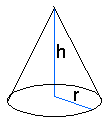Cones Volume = 1/3 pr2 x height V= 1/3 pr2h Surface = pr2 + prs S = pr2 + prs =pr2 + pr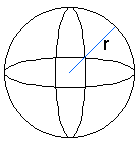Sphere Volume = 4/3 pr3 V = 4/3 pr3 Surface = 4pr2 S = 4pr2